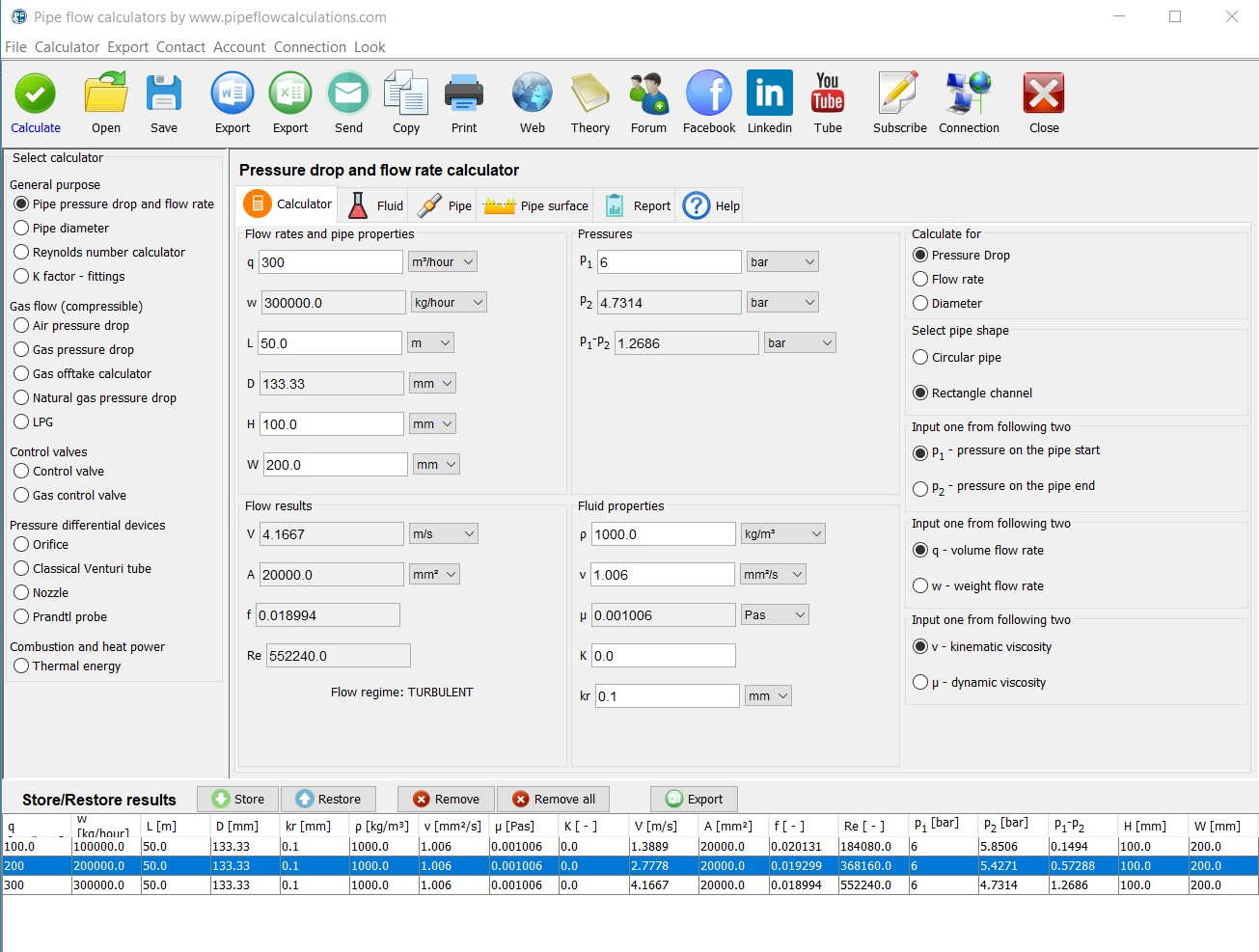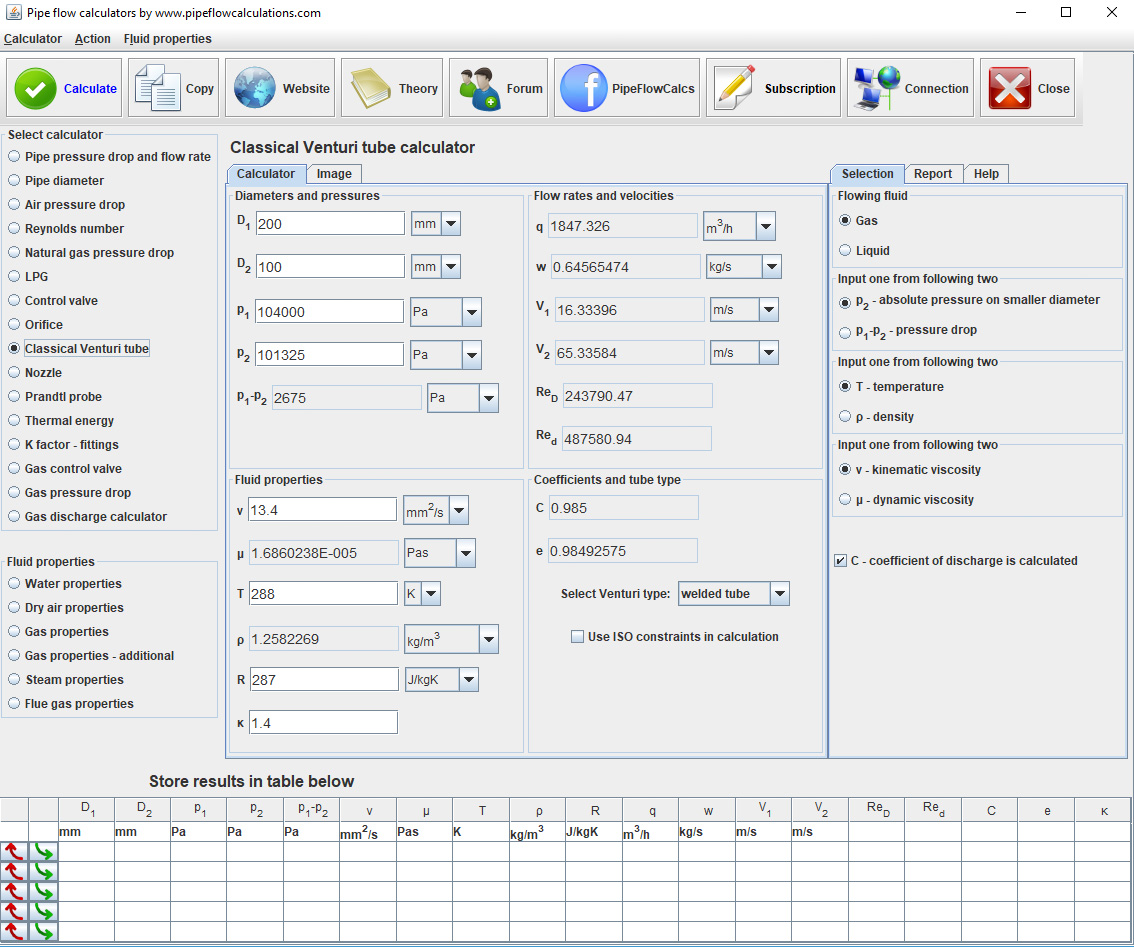# Pipe diameter and flow rate calculator

## round, closed, full pipe diameter calculation

### online since 2007## Registration and subscription

Please login for full calculator service. Use the form on the top of the page.
Register an account if you don't have one.
Active subscription is required for full services.
Action
Calculation setup
Flow rates and velocity
Pipe dimensions
Fluid properties

# Need a helping hand?If you need a quick calculation, but you are not still familiar how to use the calculator, you can order calculation service from the calculator developer.

# Calculator description

Also, read about calculation procedures, what you have to know for the calculations and what limitations apply.

# Registration and subscription

• One click switch from metric to imperial units
• Export results in word or excel format
• Preview, copy and paste results from a web page to your text editor
• Send results to you email

## Calculator start selection

Read all about available deployments. In any way of utilizing calculator, Internet connection is required, for authentication at least.

Having problems with calculators usage? Read more about known issues and problems and way to solve it.

#### Description

q - volumetric flow rate
Fluid flow rate in terms of units of volume per unit of time
ṁ - mass flow rate
Fluid flow rate in terms of units of mass per unit of time
D - pipe diameter
Internal circular pipe diameter
V - velocity
Flow velocity in terms of units of distance per unit of time
A - area
Internal pipe cross section area
ρ - fluid density
Mass per unit of volume
T - temperature
Fluid temperature for gas density calculation based on the ideal gas state equation
p - pressure
Fluid pressure at the start of the pipe for gas density calculation based on the ideal gas state equation
R - gas constant
Gas constant in terms of energy per unit of mass and temperature, for gas density calculation using ideal gas state equation

#### Calculation setup

Select value to calculate. You should enter not selected one.
D
pipe diameter
V
flow velocity
Select value to input. You should enter selected one. The other one will be calculated
q
volumetric flow rate
mass flow rate
Select value to input. You should enter selected one. The other one will be calculated. Available only if the gas is selected fluid
ρ
fluid density
T
temperature
Select fluid type
liquid
for incompressible fluids
gas
for fluids where ideal gas state equation is used. Enables selection between density and temperature to input.
Select pipe shape
round pipe
full cross section fluid flow
rectangle pipe
for rectangle channels and full cross section flow